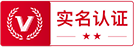﻿

# 钢筋理论重量表简介 钢筋理论重量表基本参数及计算简式

2023-02-14 13:47:45  来源：汇世网0.617是圆10钢筋每米重量。钢筋重量与直径(半径)的平方成正比。

G/m=0.617*D*D/100

Φ4=0.099Kg

Φ5=0.154Kg

Φ6=0.222Kg

Φ6.5=0.26Kg

Φ8=0.395Kg

Φ10=0.617Kg

Φ12=0.888Kg

Φ14=1.21Kg

Φ16=1.58Kg

Φ18=2.0Kg

Φ20=2.47Kg

Φ22=2.98Kg

Φ25=3.85Kg

Φ28=4.83kg

Φ30=5.549kg

Φ32=6.31kg

Φ36=7.99kg

Φ38=8.903kg

Φ40=9.87kg

Φ50=15.42kg

1、角钢、工字钢和槽钢的准确计算公式繁琐，表列简式用于计算近似值。

2、f值：一般型号及带a的为3.34，带b的为2.65，带c的为2.26。

3、e值：一般型号及带a的为3.26，带b的为2.44，带c的为2.24。

4、各长度单位均为毫米。

## 相关新闻